## Main

The back-action process considered in this paper involves deleterious inelastic tunnelling events between two adjacent dots in a serial double or triple quantum dot (DQD, TQD). The energy difference Δ between the initial and final electronic dot states is provided by the absorption of a non-equilibrium acoustic phonon, which itself is generated by the quantum point contact (QPC) detector12. Such an absorption process between adjacent dots is constrained by the energy conservation condition Δ=|q|vph (vph is the sound velocity, q the phonon wave vector). More subtly, it is also sensitive to the difference in phase, Δ φ=d · q, of the associated phonon wave between the two dot positions, with d being the vector connecting the two dot centres14,15. This q-dependent (and hence Δ-dependent) phase difference controls the matrix element for phonon absorption because it determines whether the electron–phonon couplings in each of the two individual dots add constructively or destructively (Fig. 1 and ref. 16). The result is an oscillatory probability for inelastic electron-transfer events involving phonon absorption, with constructive interference occurring when Δ φ=(2n+1)π (where n is an integer).

Data showing a pronounced back-action effect are shown in Fig. 2a, which shows the stability diagram measured in charge detection for a few-electron DQD without a voltage drop between its left and right leads. The charge configuration of the quantum-dot structures influences the conductance of a nearby QPC because of the capacitive coupling between the dots and the QPC. To serve as a charge detector it is necessary to drive a current through the detector QPC, which, in turn, leads to the observed detector back-action. Multiple gates fabricated 85 nm above a high-mobility two-dimensional electron system (2DES) are used to define two dots and two QPCs (Fig. 2d). The differential transconductance dIQPC/dVL of the biased charge detector QPC (V QPC=−1.2 mV) is plotted as a function of control gates V L,V R. It shows local extrema at the boundaries between regions of different electronic ground states, yielding dark ‘charging’ and white ‘charge transfer’ lines. Specific ground state configurations are labelled (NL,NR), where the integer Nξ denotes the number of electrons in dot ξ=L (left) and R (right). As our DQD is cooled to T30 mK the unmeasured DQD is expected to be in its ground state.

Detector back-action manifests itself within a distinct triangular-shaped region of deviations from the ground state configuration (1, 2), where a pronounced pattern of repeated, parallel stripes is present. It indicates an oscillating probability to find the DQD in the excited configuration (1, 1). The excitation process sketched in Fig. 2c includes an inelastic tunnelling transition (1,2)→(2,1) mediated by the absorption of a phonon, followed by an elastic (and therefore quick) tunnelling process (2,1)→(1,1). In our measurements, the tunnel barrier between the right dot and right lead is tuned to be almost closed (Fig. 2c,d). The direct transition (1,1)→(1,2) back into the ground state via an elastic tunnelling process from the right lead is consequently very slow and the excited configuration (1, 1) is metastable. The associated three-level dynamics can result in average non-thermal occupations13. In this way a metastable excited state is essential to directly observe detector back-action in a low-bandwidth stability diagram measurement. It requires asymmetric dot–lead tunnel couplings in the case of a DQD (Supplementary Information).

The stripe pattern constitutes the key signature of the coherent phonon-mediated back-action effect. It indicates that the probability to be in the excited configuration (1,1) oscillates as a function of the energy detuning Δ between the intermediate state (2,1) and the ground-state configuration (1,2) (Fig. 2b); each stripe is thus parallel to the white charge transfer line where these states are degenerate (that is, Δ=0; marked). The striped region is bounded by a line Δ=Δmax, indicating that there is a maximum energy available to excite the DQD. By seeing how this boundary changes with increasing V QPC (Fig. 2e,f), we find that Δmax|e V QPC|, consistent with the QPC indeed being the energy source for the initial DQD excitation.

The geometry of the back-action regions as well as the influences of temperature and the orbital excitation spectrum are discussed in the Supplementary Information. In short, the remaining boundaries of the triangular-shaped regions of back-action correspond to energy thresholds for lead tunnelling. The width of each stripe is largely independent of temperature; this is indicative of an excitation process involving electron transfers between dots, without any involvement of lead electrons (Figs 2c and 3e,f). The regular spacing of the stripe features in both DQD and TQD (discussed below) experiments over so many stripes eliminates the possibility that they are due to resonances with orbital excitations of a dot, as there is no reason to expect such a uniform level spacing; furthermore, the energy spacing between the stripes is much smaller than would be expected for the average level spacing of the small dots studied here.

To quantify the interpretation of the stripe pattern in Fig. 2 in terms of interference and QPC back-action, we have developed a theoretical model that describes the generation of phonons by the non-equilibrium QPC charge fluctuations8 and their coherent absorption by the DQD. These fluctuations represent the fundamental back-action of the measurement—their magnitude is bounded from below by the rate at which information is obtained from the QPC via a Heisenberg-like inequality8. Given this, the back-action charge noise mechanism we describe must necessarily make a contribution to the observed oscillations. This mechanism is also consistent with the high visibility of the oscillations, as such visibility requires a highly localized source of hot phonons. Although we cannot completely rule out that other, less direct, back-action mechanisms also contribute (for example, generation of hot phonons in the QPC leads), it is not clear that such mechanisms would also yield such high-visibility oscillations. We describe bulk acoustic phonon modes of GaAs interacting with both electrons in the DQD, as well as with the fluctuating charge density of the biased QPC via a screened piezoelectric interaction. Using Keldysh perturbation theory, we can calculate the DQD state in the presence of back-action (Fig. 4 and Supplementary Information). The relevant part of the dot–phonon interaction (that is, terms that can cause transitions in the dot) take the form:Here |g〉 (|e〉) denotes the DQD ground (excited) state, tc is the pertinent interdot tunnel matrix element,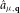(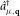) destroys (creates) a phonon of wavector q in branch μ, λq,μ is the effective matrix element (screened) for the interaction of phonons with a single dot and h.c. denotes the Hermitian conjugate. The first bracketed factor in the sum of equation (1) denotes the key interference of relevance: the two terms correspond to phonons interacting with electrons in either the left or right dot (which are centred at rL and rR respectively) (Fig. 1b).

Despite the explicit interference evident in equation (1), geometric averaging can still strongly suppress interference oscillations in observable quantities. Simply put, although the DQD ground-excited energy splitting fixes the magnitude of a phonon participating in an inelastic tunnelling event, it does not specify its direction; hence, the relative phase in the first term of equation (1) is not completely determined by Δ. This is typically the case in situations probing the emission of acoustic phonons by biased DQDs16, where interference oscillations are observed, albeit with much smaller visibilities than seen here17,18. In contrast, the simple geometric filtering depicted in Fig. 1a suggests that this averaging need not play a role in phonon absorption, as only phonons travelling from the QPC to the dots contribute. This is supported by our theoretical calculations, which also exhibit strong oscillations for realistic parameter values, and show a pronounced enhancement of interference oscillations when the DQD and QPC are all collinear (Fig. 4a–c).

The theory is also able to capture other aspects of the experimental data: in particular, the size of the back-action triangle grows with |V QPC|, and the lowest-energy stripes (that is, the smallest values of Δ corresponding to long phonon wavelengths) are suppressed because of screening effects (see Fig. 4b). Using the fact that in the experiment the QPC and DQD are approximately collinear, the measured spacing of the interference parameter δ Δ=45 μeV in the DQD data of Fig. 2d yields a DQD separation d=h vs/δ Δ250 nm; this is in good agreement with the separation estimated from scanning electron micrographs (Fig. 2d). The theory also shows that owing to the anisotropy of the electron–phonon matrix elements λq,μ, the overall magnitude of the phonon-induced back-action is sensitive to the orientation of the dot–QPC axis with respect to crystallographic axes. This dependence on orientation is demonstrated in Fig. 4d. More details on the theoretical treatment is provided in Methods and Supplementary Information.

Although we have focused so far on back-action in DQDs, the mechanism we describe is extremely general, and is in fact even more ubiquitous in systems with more than two dots. As discussed, a key requirement to see the effect is the existence of a long-lived metastable excited state. Such a situation occurs rather naturally in serial TQD structures19,20,21,22,23, as the centre dot is effectively decoupled from one of the leads whenever either one of the other two dots (left, right) is in Coulomb blockade. This directly yields a metastable excited state in which the charge of the middle dot is unable to relax. As a consequence deviations from the ground state configuration are often observed along the charging line of the centre dot20 and back-action effects occur naturally in the stability diagram. We study detector back-action in a TQD in Fig. 3 by successively increasing |e V QPC|. Already at relatively small bias |V QPC|≤300 μV (Fig. 3a,b), a triangular-shaped region of telegraph noise is observed along the central charging line20. It indicates slowly fluctuating deviations from the ground state configuration, which can be caused by external noise or detector back-action10. The underlying excitation processes, sketched in Fig. 3e,f, are similar to the one discussed above for the DQD. Indeed, the population of the right dot does not fluctuate; it plays the same role as the closed barrier in the case of the DQD, namely to block charge exchange between the centre dot and the right lead. Further increasing |V QPC| to 500 μV in (Fig. 3c) reveals the familiar pattern of equally spaced stripes, both within the (1,0,0) and (0,1,1) regions. As the bias is increased even more to |V QPC|=700 μV (Fig. 3d) the striped regions expand further, revealing the V QPC dependence also observed in the case of the DQD (Fig. 2f).

By considering experimental data on both DQD and TQD systems, we have demonstrated that interference can strongly affect the phonon-mediated back-action generated by a QPC in quantum-dot circuits. Furthermore, we have shown that this effect is well described by a basic theoretical model incorporating the generation of phonons by the QPC detector and their coherent absorption by the dots. Our study suggests the possibility of mitigating back-action effects by making use of this interference. One could, for example, endeavour to first tune the DQD/TQD to an operating point where destructive interference suppresses phonon absorption, and only then energize the QPC to make a measurement. More complex schemes that also incorporate the anisotropy of the electron–phonon interaction with respect to crystallographic axes could potentially yield even greater back-action reduction. Because the piezoelectric coupling to in-plane phonons is maximized in the 〈110〉 directions24, by aligning the QPC–DQD axis away from these directions, one could appreciably decrease the phonon-mediated back-action excitation discussed here (for example, Fig. 4a versus Fig. 4d).

## Methods

### Experiment.

The samples were fabricated from GaAs/AlGaAs heterostructures containing 2DESs 100 nm (TQD) and 85 nm (DQD) below the surface, respectively. The 2DESs are characterized at cryogenic temperatures by carrier densities of nS=2.1×1011 cm−2 and nS=1.9×1011 cm−2 with mobilities of μ=1.72×106 cm2 Vs−1 and μ=1.19×106 cm2 Vs−1 for the TQD and DQD, respectively. Metallic gate electrodes were fabricated on the sample surface by electron-beam lithography and standard evaporation/liftoff techniques. Negative voltages applied to these electrodes are used to locally deplete the 2DESs and thereby define the quantum dot and QPC structures. All measurements were performed in dilution refrigerators at cryogenic temperatures below 100 mK. To detect the charge configuration of the TQD, the voltage of one gate of the TQD was slightly modulated and the detector differential transconductance was measured in linear response (a.c. set-up). A constant voltage was also applied across the QPC to enhance detector back-action. In the case of the DQD only a constant voltage was applied across the QPC and the direct current IQPC flowing through the QPC was measured to detect the charge configuration of the DQD (d.c. set-up). The differential transconductance dIQPC/dV L was then computed numerically. Both methods result in the differential transconductance and their interpretation is identical.

To interpret the observed back-action in terms of the energy detuning Δ between different charge configurations an accurate conversion from gate voltages to units of energy is necessary. Such a linear transformation has been performed, following the methods described elsewhere25. The conversion relation reads Δ=(αgLRαgLL)V L+(αgRRαgRL)V R, with the following set of conversion factors determined for the red symbols in Fig. 2f: αgLR=(54±5) meV/V , αgRR=(105±4) meV/V , αgRL=(62±4) meV/V , αgLL=(90±5) meV/V . The conversion factors related to the blue symbols in Fig. 2f read αgLR=(65±6) meV/V , αgRR=(109±7) meV/V , αgRL=(61±6) meV/V , αgLL=(97±7) meV/V .

### Theory.

The fluctuating non-equilibrium QPC charge density operator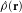is modelled as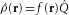, where the total charge operator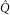is described by scattering theory (see refs 8, 26). Note that as we are interested in a single-channel QPC, the spatial profile f(r) of the fluctuating QPC charge density is fixed; for simplicity, we take it to be a Gaussian of width rQPC. This fluctuating QPC charge density is coupled to acoustic phonons via the standard piezoelectric interaction (using parameters appropriate for GaAs (ref. 24)). We calculate the Keldysh Green functions of the acoustic phonons in the presence of this coupling to the QPC, working to leading order in the electron–phonon interaction, and using scattering theory to calculate the QPC Keldysh Green functions. We then use these ‘dressed’ phonon Green functions to calculate the Fermi golden rule excitation rate of the DQD via the coupling described in equation (1). This excitation rate is finally incorporated into a master equation describing the occupation probability of the three relevant DQD states (Fig. 2c). In addition to the excitation rate (top panel of Fig. 2c), there is a rate Γfast describing the tunnelling from the excited state to the metastable auxiliary state (middle panel of Fig. 2c) and a rate Γslow describing the slow decay back to the true ground state (bottom panel of Fig. 2c). We take Γfast=1 GHz and Γslow=10 kHz; in this regime of ΓfastΓslow, the non-ground state population of the DQD is independent of Γfast, whereas Γslow determines the overall magnitude of the interference oscillations. By using the master equation to calculate the stability diagram as a function of gate voltages, one can obtain the DQD charge susceptibility d(〈nR〉+εnL〉)/dV L, which is proportional to the measured transconductance. The parameter ε0.4 characterizes the QPC’s asymmetric response to charge in the R versus L dot. Further details about the explicit form of λq,μ (including the role of screening and dimensionality) are provided in the Supplementary Information.